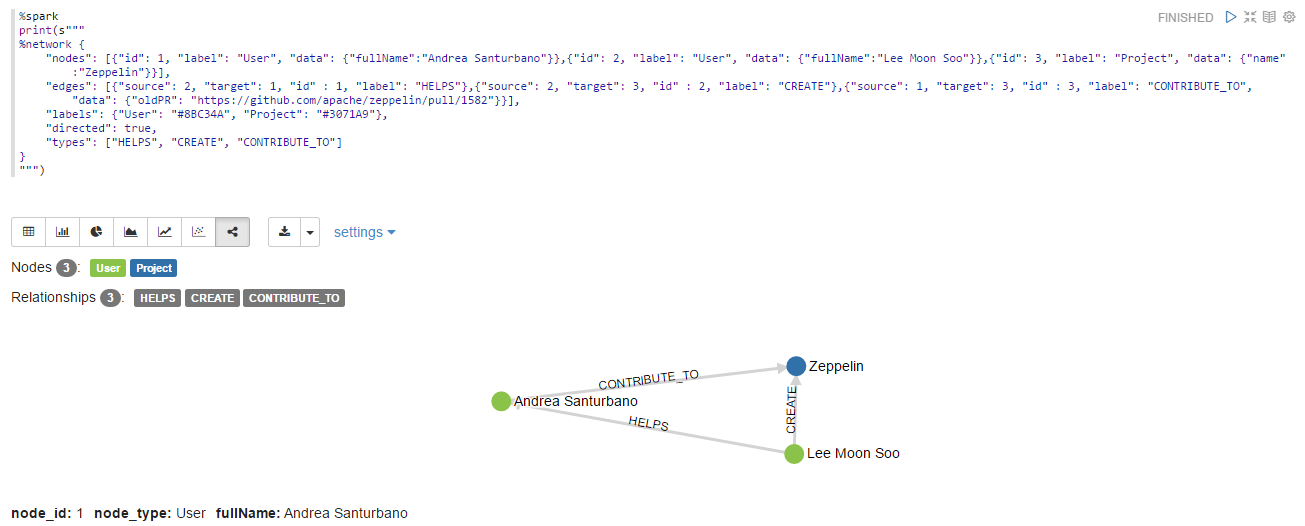# Basic Display System in Apache Zeppelin

## Text

By default, Apache Zeppelin prints interpreter response as a plain text using text display system.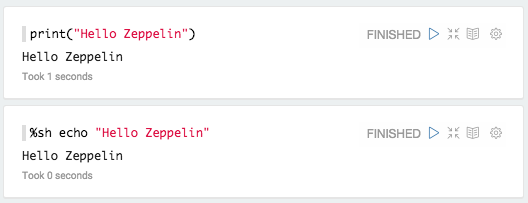You can explicitly say you're using text display system.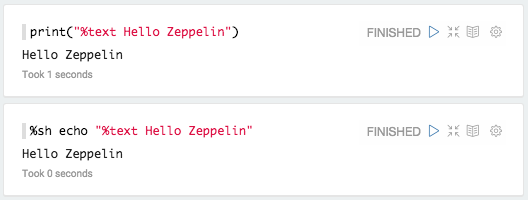## Html

With %html directive, Zeppelin treats your output as HTML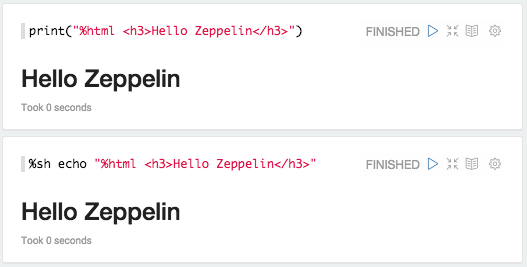### Mathematical expressions

HTML display system automatically formats mathematical expression using MathJax. You can use \$$INLINE EXPRESSION \$$ and $$EXPRESSION$$ to format. For example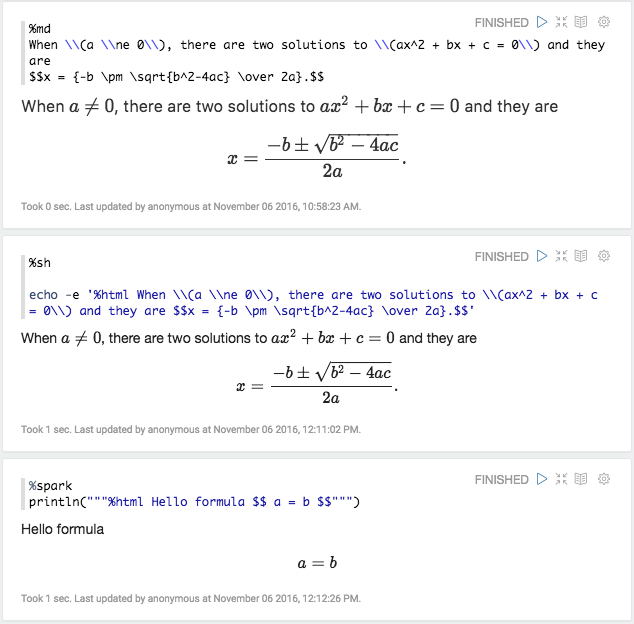## Table

If you have data that row separated by \n (newline) and column separated by \t (tab) with first row as header row, for example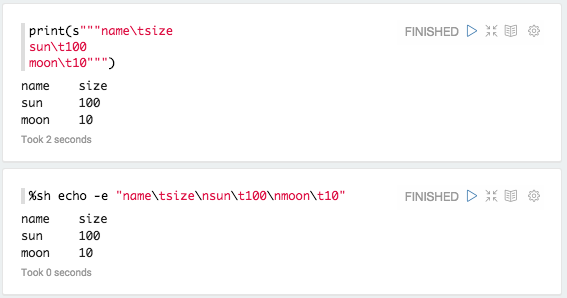You can simply use %table display system to leverage Zeppelin's built in visualization.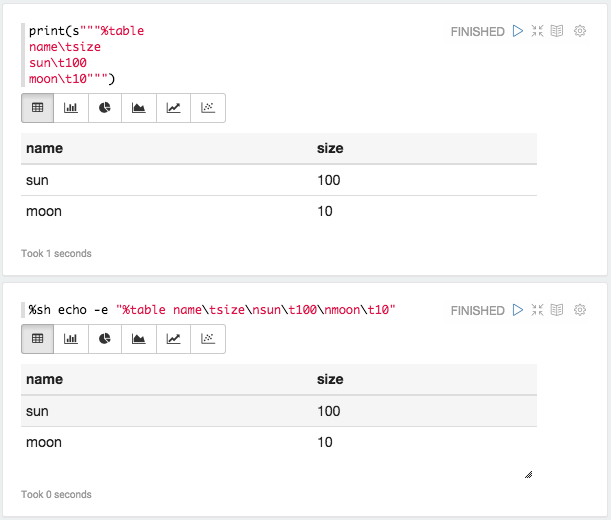If table contents start with %html, it is interpreted as an HTML.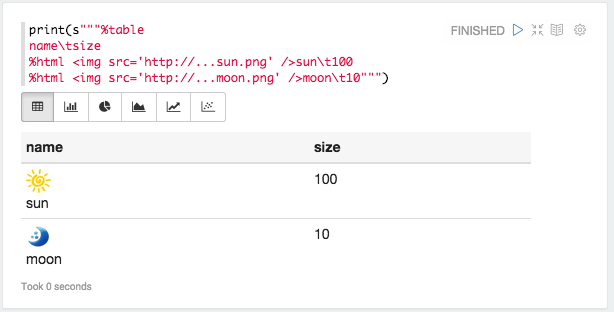Note : Display system is backend independent.

## Network

With the %network directive, Zeppelin treats your output as a graph. Zeppelin can leverage the Property Graph Model.

### What is the Labelled Property Graph Model?

A Property Graph is a graph that has these elements:

• a set of vertices
• each vertex has a unique identifier.
• each vertex has a set of outgoing edges.
• each vertex has a set of incoming edges.
• each vertex has a collection of properties defined by a map from key to value
• a set of edges
• each edge has a unique identifier.
• each edge has an outgoing tail vertex.
• each edge has an incoming head vertex.
• each edge has a label that denotes the type of relationship between its two vertices.
• each edge has a collection of properties defined by a map from key to value.A Labelled Property Graph is a Property Graph where the nodes can be tagged with labels representing their different roles in the graph model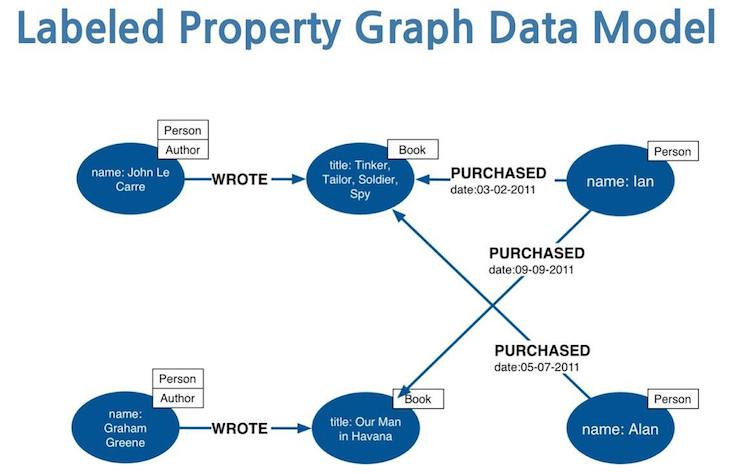### What are the APIs?

The new NETWORK visualization is based on json with the following params:

• "nodes" (mandatory): list of nodes of the graph every node can have the following params:
• "id" (mandatory): the id of the node (must be unique);
• "label": the main Label of the node;
• "labels": the list of the labels of the node;
• "data": the data attached to the node;
• "edges": list of the edges of the graph;
• "id" (mandatory): the id of the edge (must be unique);
• "source" (mandatory): the id of source node of the edge;
• "target" (mandatory): the id of target node of the edge;
• "label": the main type of the edge;
• "data": the data attached to the edge;
• "labels": a map (K, V) where K is the node label and V is the color of the node;
• "directed": (true/false, default false) wich tells if is directed graph or not;
• "types": a distinct list of the edge types of the graph

If you click on a node or edge on the bottom of the paragraph you find a list of entity properties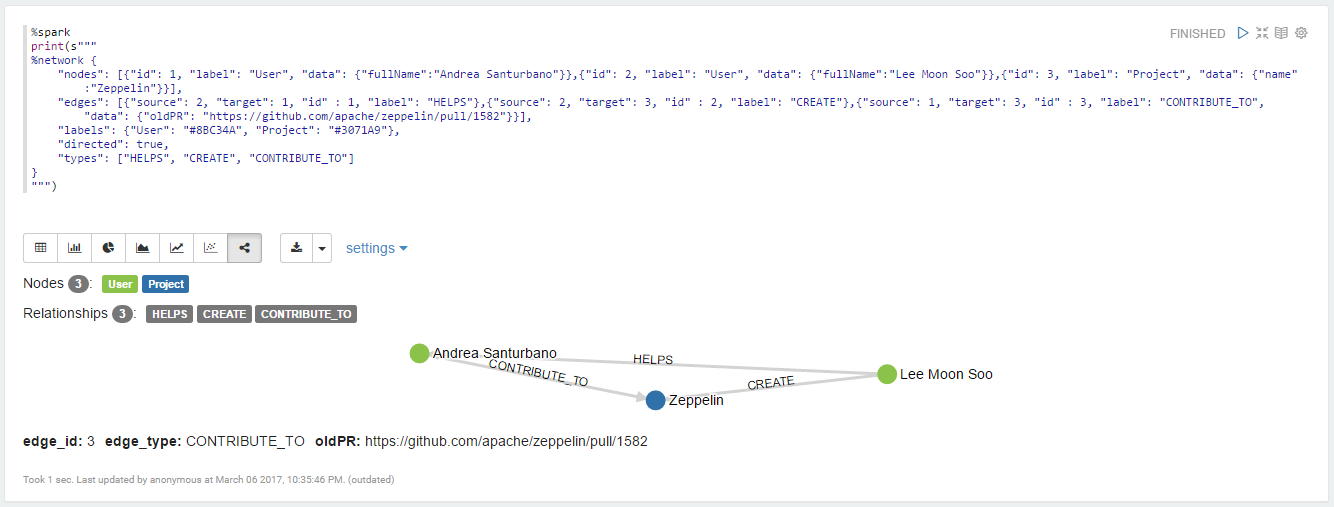This kind of graph can be easily flatten in order to support other visualization formats provided by Zeppelin.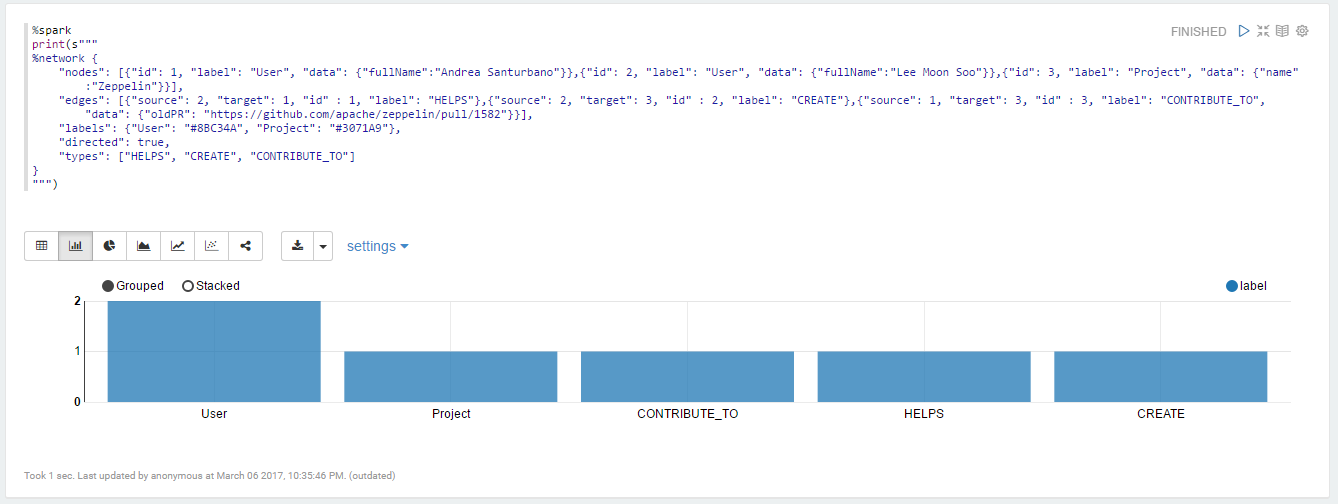### How to use it?

An example of a simple graph

%spark
print(s"""
%network {
"nodes": [
{"id": 1},
{"id": 2},
{"id": 3}
],
"edges": [
{"source": 1, "target": 2, "id" : 1},
{"source": 2, "target": 3, "id" : 2},
{"source": 1, "target": 2, "id" : 3},
{"source": 1, "target": 2, "id" : 4},
{"source": 2, "target": 1, "id" : 5},
{"source": 2, "target": 1, "id" : 6}
]
}
""")


that will look like: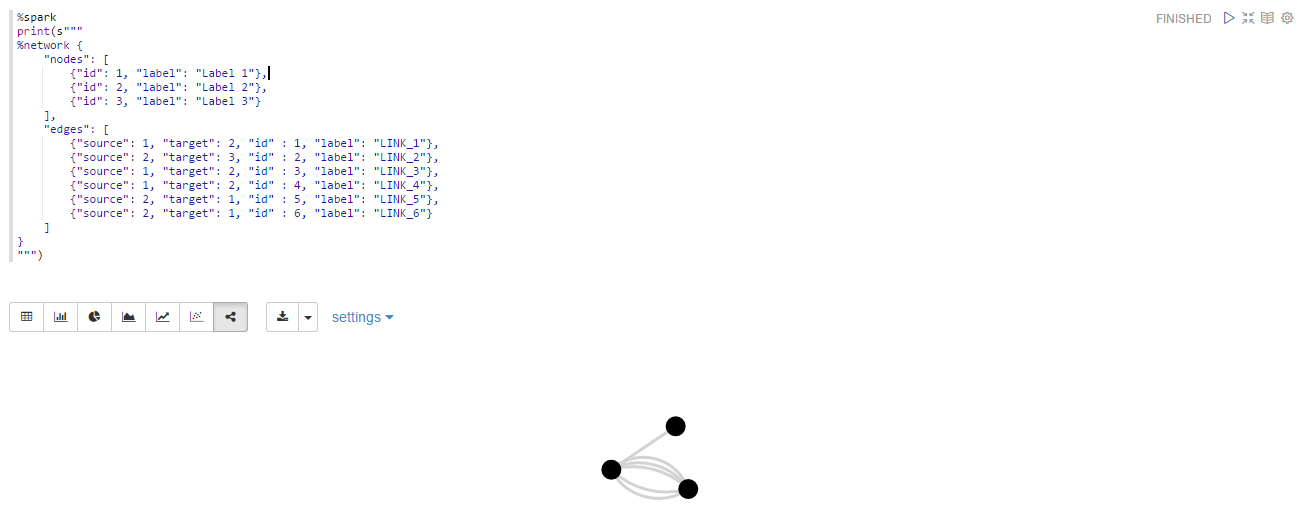A little more complex graph:

%spark
print(s"""
%network {
"nodes": [{"id": 1, "label": "User", "data": {"fullName":"Andrea Santurbano"}},{"id": 2, "label": "User", "data": {"fullName":"Lee Moon Soo"}},{"id": 3, "label": "Project", "data": {"name":"Zeppelin"}}],
"edges": [{"source": 2, "target": 1, "id" : 1, "label": "HELPS"},{"source": 2, "target": 3, "id" : 2, "label": "CREATE"},{"source": 1, "target": 3, "id" : 3, "label": "CONTRIBUTE_TO", "data": {"oldPR": "https://github.com/apache/zeppelin/pull/1582"}}],
"labels": {"User": "#8BC34A", "Project": "#3071A9"},
"directed": true,
"types": ["HELPS", "CREATE", "CONTRIBUTE_TO"]
}
""")


that will look like: